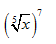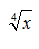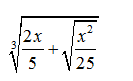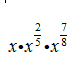N-RN Categories All categories Not categorized Modeling N-CN N-Q N-RN N-VM Prioritized

#### MAT-HS.N-RN.01

 MAT-HS Targeted Standards(N) Concept: Number and Quantity(RN) Domain: The Real Number SystemCluster: Extend the properties of exponents to rational numbers MAT-HS.N-RN.01 Explain how the definition of the meaning of rational exponents follows from extending the properties of integer exponents to those values, allowing for a notation for radicals in terms of rational exponents. For example, we define 5 1/3 to be the cube root of 5 because we want (5 1/3)3 = 5(1/3)3 to hold, so (5 1/3)3 must equal 5.

## Student Learning Targets:

• I can
• I can

• I can
• I can

### Skills (Performance) Targets

• I can use exponential properties to explain how rational exponents follow from integer exponents.

• I can
• I can

## Alg II Convert Radicals to Rational Exponents Proficiency Scale

 Score Description Sample Activity 4.0 (advanced) In addition to Score 3.0, the student demonstrates in-depth inferences and applications regarding more complex material that go beyond end of instruction expectations. 3.5 In addition to Score 3.0 performance, the student demonstrates in-depth inferences and applications regarding the more complex content with partial success. 3.0 (proficient) The student can: rewrite exponents in radical notation and radicals in exponential notation. The student exhibits no major errors or omissions. Write x2/3 in radical notation. Writein exponential notation. 2.5 The student demonstrates no major errors or omissions regarding the simpler details and processes (Score 2.0 content) and partial knowledge of the more complex ideas and processes (Score 3.0 content). 2.0 (progressing) There are no major errors or omissions regarding the simpler details and processes as the student can:  Recognize and recall specific terminology, such as: radical and radical notation index rational exponent  However, the student exhibits major errors or omissions regarding the more complex ideas and processes. Write x1/3in radical notation. Rewritein exponential notation. 1.5 The student demonstrates partial knowledge of the simpler details and processes (Score 2.0 content) but exhibits major errors or omissions regarding the more complex ideas and procedures (Score 3.0 content). 1.0 (beginning) With help, the student demonstrates a partial understanding of some of the simpler details and processes (Score 2.0 content) and some of the more complex ideas and processes (Score 3.0 content). 0.5 With help, the student demonstrates a partial understanding of some of the simpler details and processes (Score 2.0 content) but not the more complex ideas and processes (Score 3.0 content). 0.0 Even with help, the student demonstrates no understanding or skill.

## Resources

 Web Vocab rational exponent

#### MAT-HS.N-RN.02

 MAT-HS Targeted Standards(N) Concept: Number and Quantity(RN) Domain: The Real Number SystemCluster: Extend the properties of exponents to rational numbers MAT-HS.N-RN.02 Rewrite expressions involving radicals and rational exponents using the properties of exponents

## Student Learning Targets:

### Knowledge Targets

• I can understand that the denominator of a rational exponent is the root index and the numerator is the exponent of the radicand.

• I can
• I can

### Skills (Performance) Targets

• I can convert an expression in radical form to rational exponents and vice-versa.

• I can
• I can

## Alg II Re-write with Exponents Proficiency Scale

 Score Description Sample Activity 4.0 (advanced) In addition to Score 3.0, the student demonstrates in-depth inferences and applications regarding more complex material that go beyond end of instruction expectations. Simplify the following:3.5 In addition to Score 3.0 performance, the student demonstrates in-depth inferences and applications regarding the more complex content with partial success. 3.0 (proficient) The student can: apply properties of exponents to simplify expressions including rational exponents. Simplify the following expression.(41/3)3/4 (27p6)-5/3 2.5 The student demonstrates no major errors or omissions regarding the simpler details and processes (Score 2.0 content) and partial knowledge of the more complex ideas and processes (Score 3.0 content). 2.0 (progressing) There are no major errors or omissions regarding the simpler details and processes as the student can: perform operations with fractions. simplify fractions. recognize and recall specific terminology such as: exponents rational exponents However, the student exhibits major errors or omissions regarding the more complex ideas and processes. Simplify the following expressions. (p3)-2 (10/8)3 1.5 The student demonstrates partial knowledge of the simpler details and processes (Score 2.0 content) but exhibits major errors or omissions regarding the more complex ideas and procedures (Score 3.0 content). 1.0 (beginning) With help, the student demonstrates a partial understanding of some of the simpler details and processes (Score 2.0 content) and some of the more complex ideas and processes (Score 3.0 content). 0.5 With help, the student demonstrates a partial understanding of some of the simpler details and processes (Score 2.0 content) but not the more complex ideas and processes (Score 3.0 content). 0.0 Even with help, the student demonstrates no understanding or skill.

## Resources

#### MAT-HS.N-RN.03

 MAT-HS Targeted Standards(N) Concept: Number and Quantity(RN) Domain: The Real Number SystemCluster: Use properties of rational and irrational number MAT-HS.N-RN.03 Explain why the sum or product of two rational numbers is rational; that the sum of a rational number and an irrational number is irrational, and that the product of a nonzero rational number and an irrational number is irrational.

## Student Learning Targets:

### Knowledge Targets

• I can identify the properties of the real number system, i.e., commutative, associative, distributive, closure, inverse, and identity properties.

### Reasoning Targets

• I can apply the properties of the real number system to simplify an expression.

### Skills (Performance) Targets

• I can use the properties of real numbers to simplify an expression and solve an equation.
• I can show by example the sum of a rational number and an irrational number is irrational.
• I can show by example the product of a nonzero rational number and an irrational number is irrational.
• I can use the closure property or show by example the sum or product of two rational numbers is rational.

• I can
• I can

## Proficiency Scale

 Score Description Sample Activity 4.0 In addition to Score 3.0, the student demonstrates in-depth inferences and applications regarding more complex material that go beyond end of instruction expectations. - 3.5 In addition to Score 3.0 performance, the student demonstrates in-depth inferences and applications regarding the more complex content with partial success. 3.0 “The Standard.” The student demonstrates no major errors or omissions regarding any of the information and processes that were end of instruction expectations. - 2.5 The student demonstrates no major errors or omissions regarding the simpler details and processes (Score 2.0 content) and partial knowledge of the more complex ideas and processes (Score 3.0 content). 2.0 The student demonstrates no major errors or omissions regarding the simpler details and processes but exhibits major errors or omissions regarding the more complex ideas and processes (Score 3.0 content). - 1.5 The student demonstrates partial knowledge of the simpler details and processes (Score 2.0 content) but exhibits major errors or omissions regarding the more complex ideas and procedures (Score 3.0 content). 1.0 With help, the student demonstrates a partial understanding of some of the simpler details and processes (Score 2.0 content) and some of the more complex ideas and processes (Score 3.0 content). - 0.5 With help, the student demonstrates a partial understanding of some of the simpler details and processes (Score 2.0 content) but not the more complex ideas and processes (Score 3.0 content). 0.0 Even with help, the student demonstrates no understanding or skill. -

## Resources

 Web Vocab closure property irrational number rational number

#### MAT-HS.N-RN.04

 MAT-HS Targeted Standards(N) Concept: Numbers and Quantity(RN) Domain: The Real Number System MAT-HS.N-RN.04 Perform basic operations on radicals and simplify radicals to write equivalent expressions.

• I can
• I can

• I can
• I can

• I can
• I can

• I can
• I can

## Proficiency Scale

 Score Description Sample Activity 4.0 In addition to Score 3.0, the student demonstrates in-depth inferences and applications regarding more complex material that go beyond end of instruction expectations. - 3.5 In addition to Score 3.0 performance, the student demonstrates in-depth inferences and applications regarding the more complex content with partial success. 3.0 “The Standard.” The student demonstrates no major errors or omissions regarding any of the information and processes that were end of instruction expectations. - 2.5 The student demonstrates no major errors or omissions regarding the simpler details and processes (Score 2.0 content) and partial knowledge of the more complex ideas and processes (Score 3.0 content). 2.0 The student demonstrates no major errors or omissions regarding the simpler details and processes but exhibits major errors or omissions regarding the more complex ideas and processes (Score 3.0 content). - 1.5 The student demonstrates partial knowledge of the simpler details and processes (Score 2.0 content) but exhibits major errors or omissions regarding the more complex ideas and procedures (Score 3.0 content). 1.0 With help, the student demonstrates a partial understanding of some of the simpler details and processes (Score 2.0 content) and some of the more complex ideas and processes (Score 3.0 content). - 0.5 With help, the student demonstrates a partial understanding of some of the simpler details and processes (Score 2.0 content) but not the more complex ideas and processes (Score 3.0 content). 0.0 Even with help, the student demonstrates no understanding or skill. -

## Resources

 Web Vocab